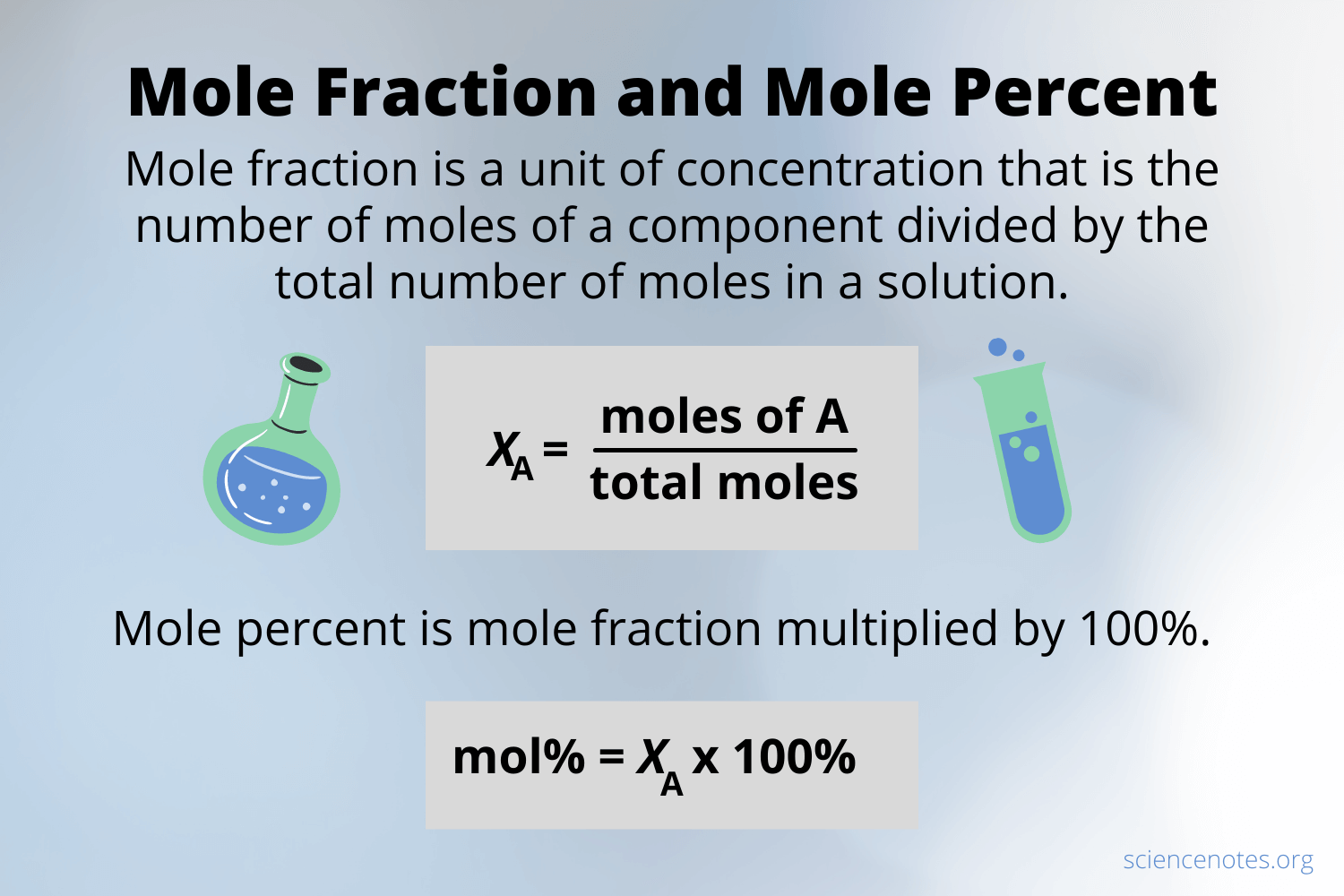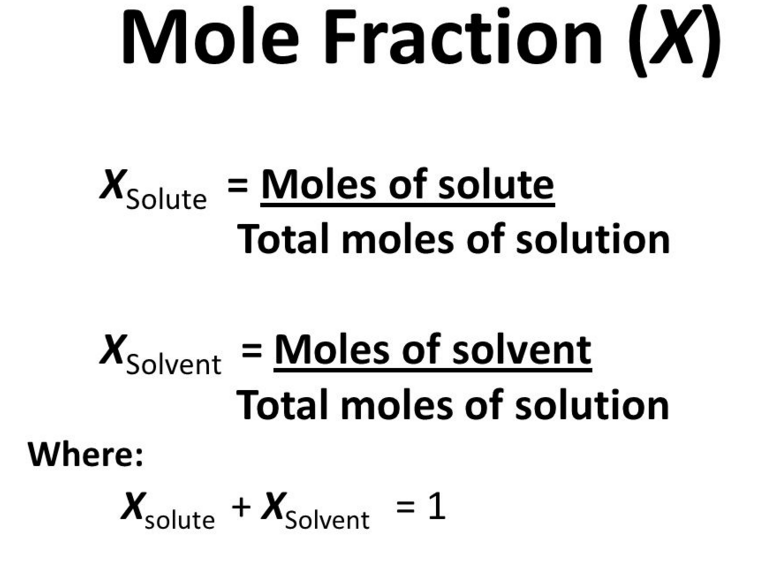# Mole Fraction Study Guide

INTRODUCTION

There is nothing as satisfying as perfectly cooked pasta. Everyone knows what ingredients are used for cooking pasta, but is it enough? The perfection of the dish lies in the concentration (what and how much) of the ingredients used. From the beginnings of chemistry to the most complex methods, concentration and its consequences are essential.Concentration is crucial from a conceptual standpoint. It informs you how much you possess and what quantity you have. Without the right concentrations, a chemical reaction that requires specific amounts of reagents may simply not function. Concentration is directly related to determining information such as the amount of heavy metal in freshwater, the amount of insecticide that has run off into a neighboring water source, and the severity of acid rain.

### WHAT IS THE MOLE FRACTION?

The mole fraction is one of the numerous methods for indicating the concentration of a solution. A mole fraction is a concentration unit. The mole fraction, symbolized by “X,” determines the relative concentration of solutes and solvents in a solution.

For a mixture of two substances, C and D, the mole fraction equation of each would be written as follows:

Xc = mol C / mol C + mol D

Xd = mol D / mol C + mol D

Mole fractions have no dimensions hence the total of all mole fractions in a combination is always 1.### MOLE FRACTION EXAMPLE

If 0.010 moles of NaCl are dissolved in 100 grams of pure water, compute the mole fraction of NaCl and H₂O.

We know, the molecular weight of water is 18.0153

• Number of moles of water = 100/18.015= 5.56 moles
• Mole fraction of NaCl = 0.10/ 5.56 + 0.100 = 0.018
• Mole fraction of H₂O = 5.56/5.66 = 0.982
• Sum of mole fractions here is 0.982 + 0.018 = 1

In conjunction with Dalton’s law of partial pressures, a mole fraction is a helpful number for studying gas mixtures. Think about the following scenario:

A 1.5 atm total pressure is exerted on the container walls by hydrogen and oxygen gas mixture. Find the partial pressure of oxygen and hydrogen in the mixture if the mole fraction of hydrogen is 0.67.

Make a list of the known quantities and devise a strategy for solving the problem.

• Total pressure = 1.5 atm

• Mole fraction of oxygen = 0.33

• Mole fraction of hydrogen = 0.67Unknown

• The partial pressure of oxygen (Po)

• The partial pressure of hydrogen (Ph)

• Now, multiplying the mole fraction by the total pressure yields the partial pressure.

• Ph = 0.67 × 1.5 = 1 atm and Po = 0.33 × 1.5 = 0.5 atm

### PROPERTIES OF MOLE FRACTION

• It is not necessary to know the densities of the states of the elements involved.
• The mole fraction of an ideal gas mixture can be calculated as the ratio of partial pressure to total pressure. This scale is symmetrical.
• A mixture of known mole fractions can be created by measuring the right masses of the elements.
• Temperature has no effect on a mole fraction. In contrast to molar concentration, mole fraction does not require knowledge of the densities of the phases involved.
• As the measure is symmetric (x=0.1 and x=0.9), the roles of ‘solute’ and ‘solvent’ are reversible in a mole fraction.
• The mole express can be represented as a function component in a ternary mixture.

The molar fraction has several advantages and distinguishing characteristics. There are no mole fraction units in a ratio; the advantages of this can be discussed.

• The molar fraction is temperature independent. Because mass is unaffected by temperature, the number of particles is unaffected. As a result, no temperature change will occur during any experiment.
• Molality is defined as the number of moles of a solute present in one kilogram of a solvent. You can easily find out the molality by converting it into kilogram’s using the ratio and what is mole fraction.
• The molar fraction makes it very simple to calculate the mole percent. You can easily calculate the mole percent by multiplying the fraction by 100. You can also get the molar fraction by dividing the mole percent by 100.
• The molar fraction can be used to determine the concentration of any solute in any given solution.
• The molar fraction can be used to determine whether pressure is rising or falling. The molar fraction changes as a result of pressure variations.
• The molar fraction is always measured symmetrically. This won’t ever alter. If the solute’s molar fraction is 0.25, the solvent’s molar fraction will be 0.95. Since the sum of all the molar fractions is a unit, these circumstances won’t alter.
• Volume Fraction and Molar are the same for perfect gas mixtures, there is no need to calculate the molar fraction and volume fraction separately.

### CONCLUSION

• A mole fraction is a concentration unit symbolized by “X.”

• Mole fractions have no dimensions hence the total of all mole fractions in a combination is always 1.

• For a mixture of two substances, C and D, the mole fraction equation of each would be written as follows:

``                   Xc = mol C / mol C + mol D                   Xd = mol D / mol C + mol D``

### FAQs

1. What is the mole fraction formula?

The formula of mole fraction is the ratio of the moles of solute/ solvent to the total moles of solute and solvent.

2. What is meant by mole fraction?

Mole fraction is a concentration unit that determines the relative concentration of solutes and solvents in a solution.

We hope you enjoyed studying this lesson and learned something cool about the Mole Fraction! Join our Discord community to get any questions you may have answered and to engage with other students just like you! Don’t forget to download our App to experience our fun VR classrooms – we promise, it makes studying much more fun! 😎

]]>